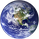7723 visualizzazioni
These Bollinger Bands are exponential because the variance is calculated using the exponential moving average , rather than just adding the normal standard deviation to the ema . This may be more useful because the exponential standard deviation should be more sensitive to near term increases or decreases in volatility .

Please do not forget that Bollinger Bands should always be combined with another method of analysis. Bollinger Bands just provide an easy way to gauge where the price could range in. At 2 standard deviations of a continuously random variable, more than 98% of data points are in this range. I am however going to test this in excel to get the average number of data points that stay in the range for Bitcoin . I will upload my findings when I complete that. Please monitor this description if your interested.
```study("Exponential Bollinger Bands", shorttitle = "EBB", overlay = true)
src = input(ohlc4, title = "source")
len = input(21, title = "timeframe / # of period's")
e = ema(src,len)
evar = (src - e)*(src - e)
evar2 = (sum(evar,len))/len
std = sqrt(evar2)
Multiplier = input(2, minval = 0.01, title = "# of STDEV's")
upband = e + (Multiplier * std)
dnband = e - (Multiplier * std)
//stdd = stdev(std)
//bsu = upband + std
//bsun = upband - std
//bsd = dnband + std
//bsdn = dnband - std
//plot(bsu, color = purple)
//plot(bsun, color = purple)
//plot(bsd, color = purple)
//plot(bsdn, color = purple)
plot(e, color = purple, linewidth = 2, title = "basis")
plot(upband, color = red, linewidth = 2, title = "up band")
plot(dnband, color = green, linewidth  = 2, title = "down band")```

## CommentiCiccioBenzina
Other platforms may be calculating the "sample standard deviation". The sample standard deviation is SIGMA((yi - ybar)^2) / (n - 1) . In this script, I am calculating the standard deviation as "population". This may be why you are seeing the in-congruence.
RispondiCiccioBenzina
You should be able to use this on whatever you want?
RispondiThanks for all the likes guys! I'm glad you all like the script!
Rispondi